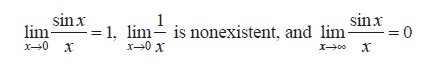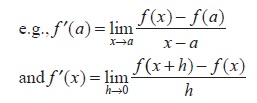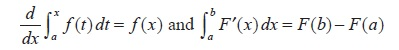beginning of content:

## Add Study Guides

### 2021 CLEP Official Study Guide

Book \$24.99

This study guide provides practice questions for all 34 CLEP exams. The ideal resource for taking more than one exam. Offered only by the College Board.

### CLEP® Calculus Examination Guide

PDF Document \$10.00

The Calculus exam is approximately 60% limits and differential calculus and 40% integral calculus.

Skip to shopping cart

# Calculus

## Overview

The Calculus examination covers skills and concepts that are usually taught in a one-semester college course in calculus. The content of each examination is approximately 60% limits and differential calculus and 40% integral calculus. Algebraic, trigonometric, exponential, logarithmic, and general functions are included. The exam is primarily concerned with an intuitive understanding of calculus and experience with its methods and applications. Knowledge of preparatory mathematics is assumed, including algebra, geometry, trigonometry, and analytic geometry.

## Knowledge and Skills Required

The examination contains 44 questions, in two sections, to be answered in approximately 90 minutes. Any time candidates spend on tutorials and providing personal information is in addition to the actual testing time.

• Section 1: approximately 27 questions, approximately 50 minutes.
No calculator is allowed for this section.
• Section 2: approximately 17 questions, approximately 40 minutes.
The use of an online graphing calculator (non-CAS) is allowed for this section. Only some of the questions will require the use of the calculator.

### Graphing Calculator

A graphing calculator, the TI-84 Plus CE, is integrated into the exam software, and available to students during Section 2 of the exam. Only some of the questions actually require the graphing calculator.

To use the calculator during the exam, students need to select the Calculator icon. Information about how to use the calculator is available in the Help icon under the Calculator tab. Students are expected to know how and when to make appropriate use of the calculator.

Information about the graphing calculator, including opportunities to practice, is available here.

In order to answer some of the questions in section 2 of the exam, students may be required to use the online graphing calculator in the following ways:

• Perform calculations (e.g., exponents, roots, trigonometric values, logarithms)
• Graph functions and analyze the graphs
• Find zeros of functions
• Find points of intersection of graphs of functions
• Find minima/maxima of functions
• Find numerical solutions to equations
• Generate a table of values for a function

Questions on the exam require candidates to demonstrate the following abilities:

• Solving routine problems involving the techniques of calculus (approximately 50% of the exam)
• Solving nonroutine problems involving an understanding of the concepts and applications of calculus (approximately 50% of the exam)

The subject matter of the Calculus exam is drawn from the following topics. The percentages next to the main topics indicate the approximate percentage of exam questions on that topic.

### Limits (10%)

• Statement of properties, e.g., limit of a constant, sum, product or quotient
• Limit calculations, including limits involving infinity, e.g.• Continuity

### ​Differential Calculus (50%)

The Derivative

• Definitions of the derivative• Derivatives of elementary functions
• Derivatives of sums, products and quotients (including tan x and cot x)
• Derivative of a composite function (chain rule), e.g., sin(ax  + b), aekx, ln(kx)
• Implicit differentiation
• Derivative of the inverse of a function (including arcsin x and arctan x)
• Higher order derivatives
• Corresponding characteristics of graphs of ƒ, ƒ′ and ƒ
• Statement of the Mean Value Theorem; applications and graphical illustrations
• Relation between differentiability and continuity
• Use of L'Hospital's Rule (quotient and indeterminate forms)

Applications of the Derivative

• Slope of a curve at a point
• Tangent lines and linear approximation
• Curve sketching: increasing and decreasing functions; relative and absolute maximum and minimum points; concavity; points of inflection
• Extreme value problems
• Velocity and acceleration of a particle moving along a line
• Average and instantaneous rates of change
• Related rates of change

### Integral Calculus (40%)

Antiderivatives and Techniques of Integration

• Concept of antiderivatives
• Basic integration formulas
• Integration by substitution (use of identities, change of variable)

Applications of Antiderivatives

• Distance and velocity from acceleration with initial conditions
• Solutions of y = ky and applications to growth and decay

The Definite Integral

• Definition of the definite integral as the limit of a sequence of Riemann sums and approximations of the definite integral using areas of rectangles
• Properties of the definite integral
• The Fundamental Theorem:Applications of the Definite Integral

• Average value of a function on an interval
• Area, including area between curves
• Other (e.g., accumulated change from a rate of change)

Notes and Reference Information

1. Figures that accompany questions are intended to provide information useful in answering the questions. All figures lie in a plane unless otherwise indicated. The figures are drawn as accurately as possible EXCEPT when it is stated in a specific question that the figure is not drawn to scale. Straight lines and smooth curves may appear slightly jagged.
2. Unless otherwise specified, all angles are measured in radians, and all numbers used are real numbers.
3. Unless otherwise specified, the domain of any function f is assumed to be the set of all real numbers x for which f ( x ) is a real number. The range of f is assumed to be the set of all real numbers f ( x ) where x is in the domain of f .
4. In this test, ln x denotes the natural logarithm of x (that is, the logarithm to the base e).
5. The inverse of a trigonometric function f may be indicated using the inverse function notation f-1 or with the prefix “arc” (e.g., sin-1 x = arcsin x)

## Study Resources

To prepare for the Calculus exam, you should study the contents of at least one introductory college-level calculus textbook, which you can find in most college bookstores.You would do well to consult several textbooks, because the approaches to certain topics may vary. When selecting a textbook, check the table of contents against the knowledge and skills required for this exam.

### Textbooks

A survey conducted by CLEP found that the following textbooks are among those used by college faculty who teach the equivalent course. You might purchase one or more of these online or at your local college bookstore.

• Anton et al., Calculus: Early Transcendentals Single Variable (Wiley)
• Armstrong and Davis, Brief Calculus (Prentice Hall)
• Bear, Understanding Calculus (Wiley-IEEE)
• Best et al., Calculus: Concepts & Calculators (Venture)
• Cohen and Henle, Calculus: The Language of Change (Jones & Bartlett)
• Hallett et al., Applied Calculus (Wiley)
• Hass et al., University Calculus, Part One (Addison-Wesley)
• Krantz, Calculus Demystified: A Self-Teaching Guide (McGraw-Hill)
• Larson et al., Calculus I: Early Transcendental Functions (Brooks/Cole)
• Neill, Teach Yourself Calculus (McGraw-Hill)
• Rogawski, Calculus (W. H. Freeman)
• Salas et al., Calculus: One Variable (Wiley)
• Schmidt, Life of Fred: Calculus (Polka Dot)
• Smith and Minton, Calculus, Single Variable: Early Transcendental Functions (McGraw-Hill)
• Stewart, Single Variable Calculus (Brooks/Cole)

### Online Resources

These resources, compiled by the CLEP test development committee and staff members, may help you study for your exam. However, none of these sources are designed specifically to provide preparation for a CLEP exam. The College Board has no control over their content and cannot vouch for accuracy.

## Score Information

Passing Score for Calculus

ACE Recommended Score*: 50
Semester Hours: 4

Each institution reserves the right to set its own credit-granting policy, which may differ from that of ACE. Contact your college as soon as possible to find out the score it requires to grant credit, the number of credit hours granted, and the course(s) that can be bypassed with a satisfactory score.

*The American Council on Education’s College Credit Recommendation Service (ACE CREDIT) has evaluated CLEP processes and procedures for developing, administering, and scoring the exams. The score listed above is equivalent to a grade of C in the corresponding course. The American Council on Education, the major coordinating body for all the nation’s higher education institutions, seeks to provide leadership and a unifying voice on key higher education issues and to influence public policy through advocacy, research, and program initiatives. Visit the ACE CREDIT website for more information.